By Jeffrey MacCormack and Ian Matheson

Two trains depart simultaneously from cities 120 kilometres apart. The first train is travelling at 40 kilometres per hour and the second train is traveling at 60 kilometres per hour. How many minutes until they collide?If you have had to answer a word problem like this one, you may have felt some anxiety as you tried to make sense of the question and choose a strategy to solve it. It may not be a surprise to you that solving word problems can be a complex task for many students. For students with learning disabilities (LDs), word problems can be particularly difficult.

Students with LDs are more likely than typically developing students to have a hard time conceptualizing and deciding on an appropriate strategy when solving problems (van Garderen, 2007). Additionally, students with LDs may choose the less successful “solution strategies” (e.g., trial and error) rather than representation strategies (e.g., diagramming; van Garderen, Scheuermann, & Jackson, 2012). For all students, one of the most effective strategies for solving word problems is to develop diagrams or, as they are known in the literature, representations (van Garderen & Montague, 2003).

## Part 1: How Diagrams can Help

Generating diagrams to solve math problems can help learners in numerous ways (Stylianou, 2010). Early in the process, diagrams can be used as a tool for recording information about the problem during the solution process. As the student starts to conceptualize the problem, diagrams can be a tool for exploring alternative ways of understanding the problem. Even as the solution is found, diagrams can be used to monitor and evaluate the solution.

Despite their usefulness, generating diagrams can be a challenging process with many steps (van Garderen, Scheuermann, & Poch, 2014). Students must decode verbal information and translate it into visual information—a process that requires students to identify and synthesize information before connecting it to prior knowledge. Next, students must identify an appropriate form of diagram based on the type of word problem. After selecting the type of diagram to be used, students must produce diagrams accurate to the content of the word problem. In more complex word problems, students may even have to modify the original diagram to incorporate new information. This process can be cognitively exhausting, and therefore extra challenging for students with LDs that possess cognitive deficits (Rosenzweig, Krawec, & Montague, 2011).

Click here to access the LD@school articles on working memory and LDs.

Click here to access the LD@school articles on Executive Function & LDs.

Proficiency in diagrams requires skills in five distinct strands (van Garderen et al., 2014):

1. Conceptual understanding involves the understanding of what a diagram is and how it can be used.
2. Procedural fluency involves generating a diagram that represents a problem.
3. Strategic competence involves using a diagram to represent, solve, and monitor progress on a problem.
4. Adaptive reasoning involves justifying how the diagram was useful in solving the problem.
5. Lastly, productive disposition involves recognizing the value of diagrams to solve problems and having the confidence to use them when appropriate.

### Students with LDs and Use of Diagrams

Students with LDs use diagrams to solve word problems in mathematics less effectively than their peers (van Garderen et al., 2012). Further, the diagrams students with LDs generate appear to be of poorer quality than those of their peers, and students with LDs use diagrams in fewer ways than their peers (van Garderen & Montague, 2003). Fortunately, research has shown that, when explicitly taught to create and use them, students with LDs are capable of learning representation strategies, like diagrams (e.g., Hutchinson, 1986).

While the use of diagrams is an effective strategy to solve word problems for students with LDs, implementing the strategy brings its own challenges. If students have poor procedural and conceptual knowledge, they may have a more difficult time creating and using meaningful diagrams (van Garderen & Scheuermann, 2015). Additionally, students need to use executive functions to focus attention and ignore extraneous details when making a diagram (Uesaka & Manalo, 2012). These processes are effortful and, as with all strategies, if the perceived relative gain of diagrams is not worth the cost, students will avoid using them (Uesaka & Manalo, 2012; van Garderen & Scheuermann, 2015).

The fundamental problem for all students, and especially those with LDs, is that making effective diagrams is difficult. Before students can design a diagram, they have to be able to visualize the problem—a developmental skill that typically matures between the ages 8 and 11 (van Garderen & Montague, 2003). Even when students are able to visualize the problem, the translation of that visualization to external images (e.g., diagramming on paper) is problematic. Students may also fail to record the information effectively because they focus on extraneous details, mislabel the diagram, or exclude important information. Most problematically, students may think that diagrams are simply visual representations of the problem and not understand that diagrams display the relational and quantitative information of the problem.

## Part 2: How to Teach Students to Use Diagrams to Solve Problems

According to van Garderen and Scheuermann (2015), there are two distinct phases to teaching students how to use diagrams to solve word problems. Before learning how to use diagrams to solve problems, students need to learn how to create diagrams. Students have to know that, as discussed earlier, effective diagrams require more than visual representations of the objects present in the word problem. Without direct instruction on diagramming, students may not fully comprehend how diagrams can be used, a competence known as meta-representation. Meta-representational competence is the student’s ability to apply knowledge about diagrams to appropriately select, produce, and use diagrams for math problems (van Garderen, Scheuermann, & Jackson, 2012).

To help students to develop their meta-representational competence, teachers should teach (a) what diagrams are, (b) why diagrams are used, (c) when a diagram should be used, (d) which type of diagram is appropriate for the math problem, (e) how to create a diagram, and (f) how to use a diagram. It is important for students to have broad conception of diagrams because while some problems naturally lend themselves to diagramming, not all problems do. For example, students use diagrams more often when solving word problems related to length or distance than when solving less spatial problems (Uesaka & Manalo, 2012). Direct instruction for diagramming is important for students with LDs because diagrams should be an integrated component of the process of solving the problem, not as a final step (van Garderen, 2007).

Students need to learn that diagrams are more than simply drawings. Ineffective diagrams, known in the literature as pictorial diagrams, depict the visual appearance of variables in the word problem (e.g., a drawing of a character in a word problem; e.g., van Garderen & Montague, 2003). Effective diagrams (known as schematic diagrams) go beyond visualizing the objects in the problem; they represent the content of the problem and depict the relational information (van Garderen, 2007). Schematic diagrams are extremely helpful for word problems in math and can be transferred across topics in mathematics, including geometry and probability, and across grades (Zahner & Corter, 2010).

Once students understand that diagrams are cognitive tools that stand in the place of, and reflect, a thinking process, they need to learn how to use them to solve math problems. During the second phase, students need to learn that using diagrams requires a three-step process:

(a) Ask: focus on what needs to be done,

(b) Do: act and/or produce, and

(c) Check: confirm the diagram helps solve the problem.

While these steps appear linear, students need to know that these steps are iterative (van Garderen & Scheuermann, 2015).

## Part 3: Examples of Pictorial and Schematic Diagrams

The following four word problems are included to illustrate the differences between pictorial and schematic diagrams. The diagrams below also demonstrate how diagrams do more than visualize the problem; diagrams are tools for solving the problem.

The comic store is 4.4 kilometres west from Vera’s house. The video game store is 2.8 kilometres west from Vera’s house. How far is the comic store from the video game store?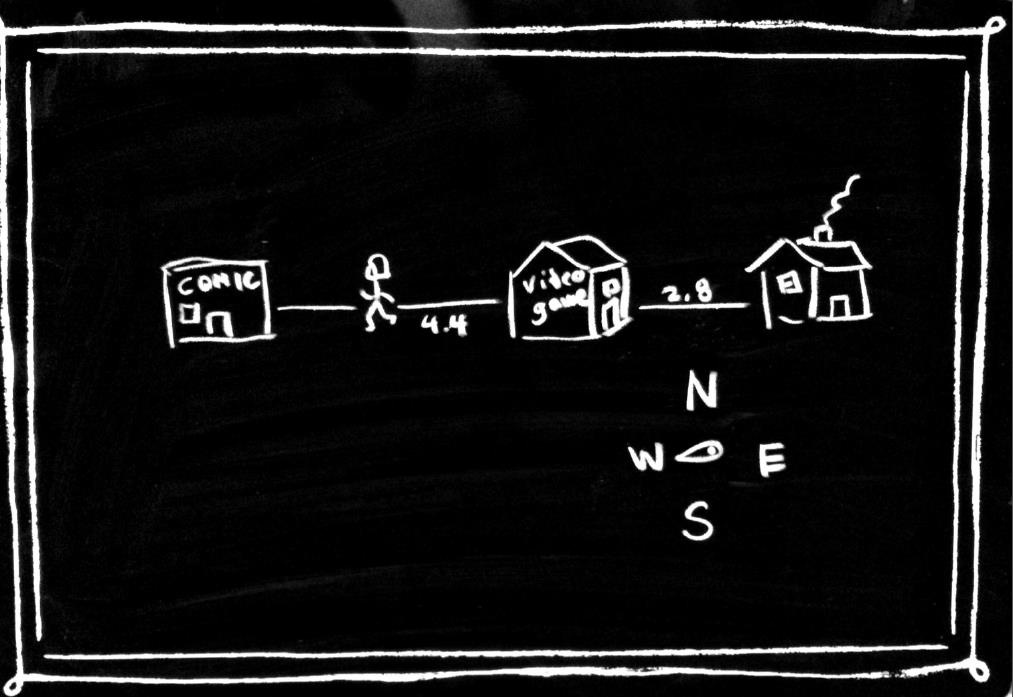Pictorial

Students with LDs may make the mistake of focusing too much attention on the details of the drawings. This student may have spent too much time drawing the buildings and including extraneous information, such as the compass. The unnecessary attention to detail may have been why the student mislabelled the distances.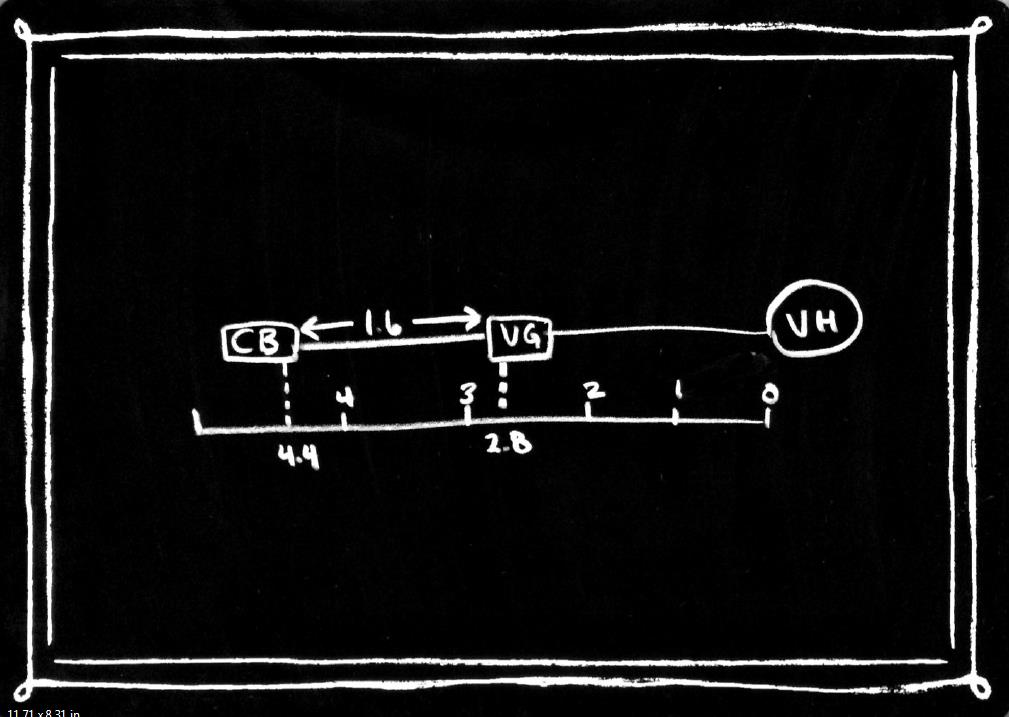Schematic

This student used a diagram that includes measures of length. By including relational information such as the distance on the number line, this student was better able to conceptualize and solve the problem.

While preparing five pizza-bagels for her four friends and herself, Leyla accidentally burned two pizza-bagels. Now they have to share the remaining pizza-bagels. What is the largest amount of pizza-bagel each person can eat if they all have the same amount?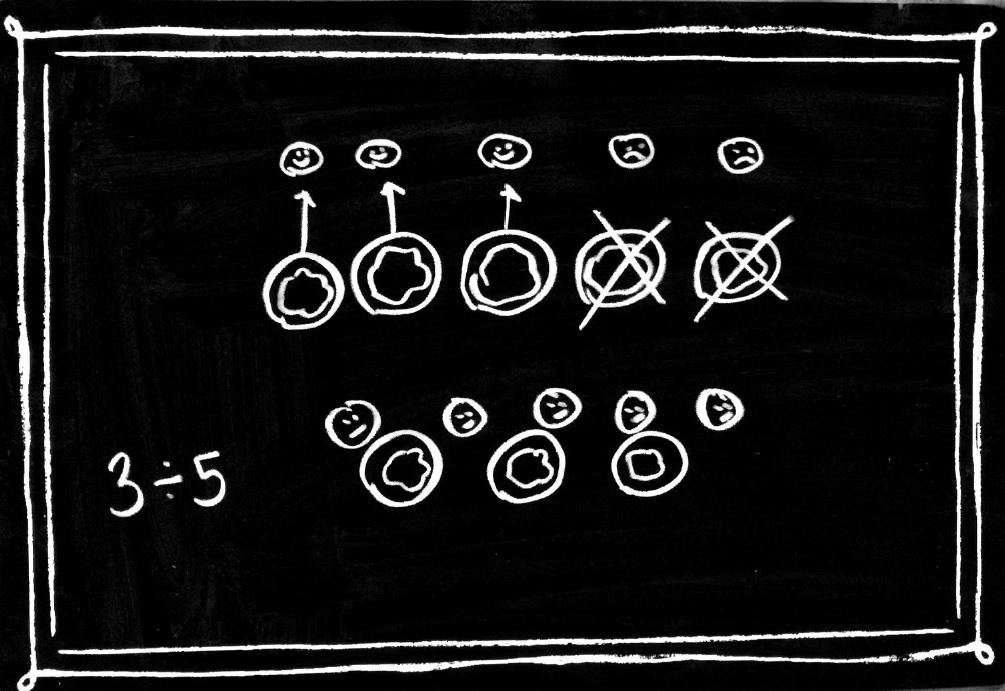Pictorial

Students are more likely to intuitively use diagrams to solve certain types of problems. For example, word problems that involve distance (see previous word problem) and sharing wholes (this question) are easier to imagine because they involve concrete relationships and amounts. Unfortunately, when students with LDs try to diagram the problem they tend to only go as far as drawing the objects in the problem. This student knew that the question required him to divide three objects among five people, but did not know how to use the diagram to forward his thinking.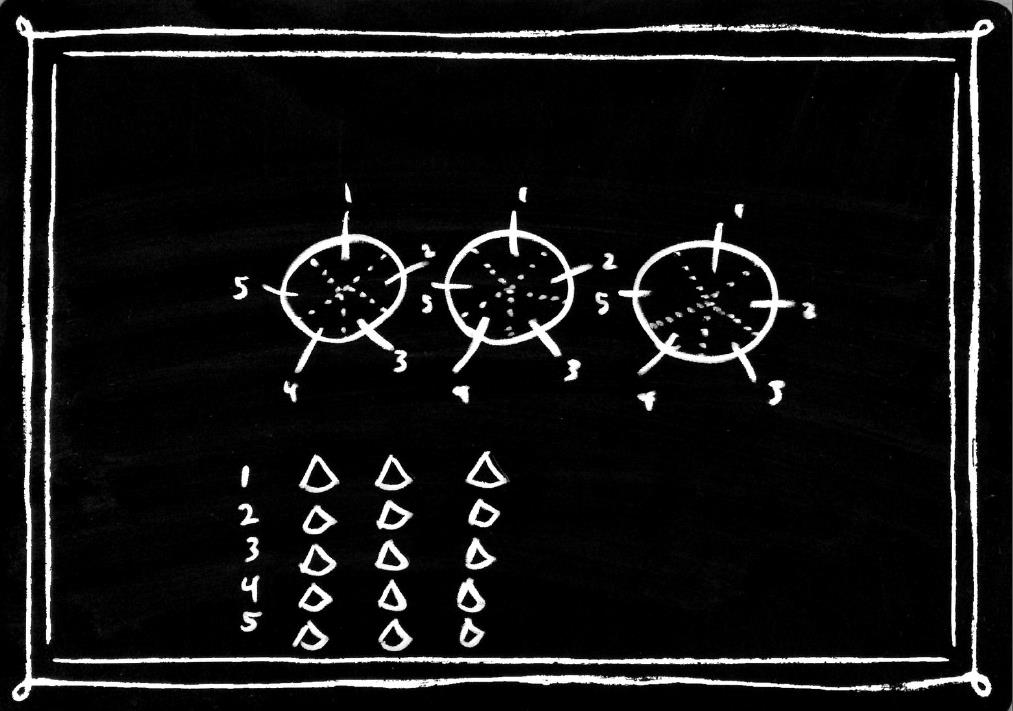Schematic

Even if students are less able to conceptualize that three bagels can be split into fifths, they may be able to imagine five friends all pulling on the same bagel at the same time. By using a diagram to illustrate friends sharing each bagel, the student may realize that, when shared equally, each friend will take one-fifth from each of the three bagels, for a total of three fifths. It is also important to note that by not drawing the faces of Leyla and her friends, the student was able to exclude visual information that was not necessary for the problem solving. Instead of drawing faces, the student used numbers (1-5) to represent the friends, thereby simplifying the visualization.

Last month, the pet store sold four times more kittens than lizards. If the pet store had sold 18 additional lizards last month, the number of kittens and lizards sold would have been equal. How many kittens were sold last month?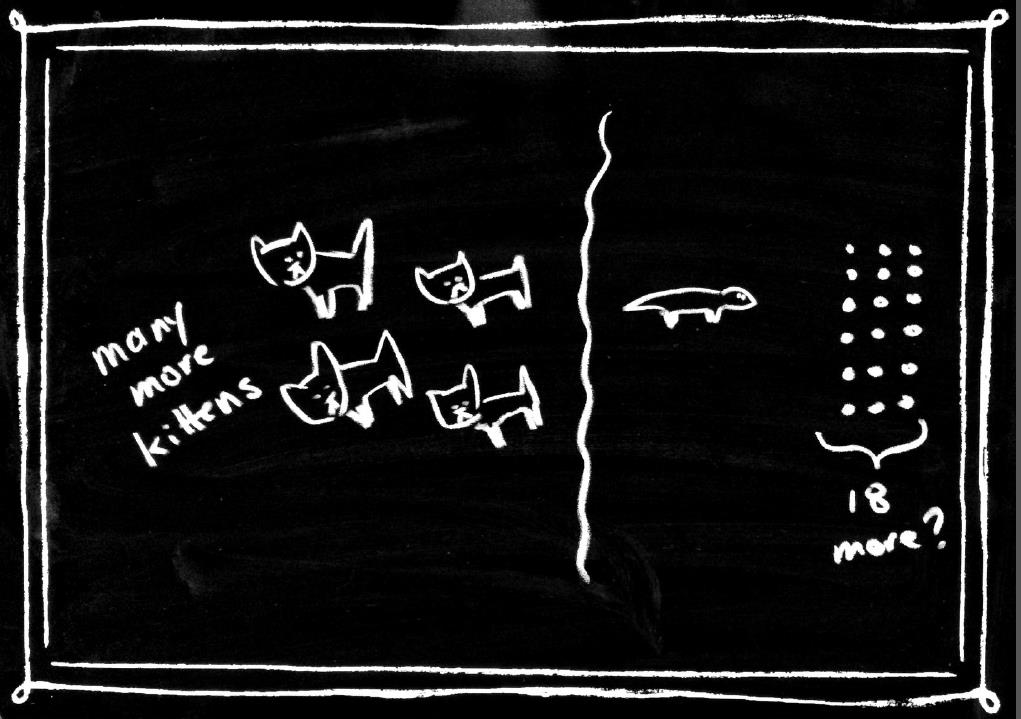Pictorial

Diagramming word problems that involve ratios are generally more difficult for students than word problems about distances. It may not always be clear how to include relational information. While this student was able to visualize that one lizard was sold for every four kittens sold, he was not able to determine how that information was related to the additional 18 lizards that would have equalled the quantities.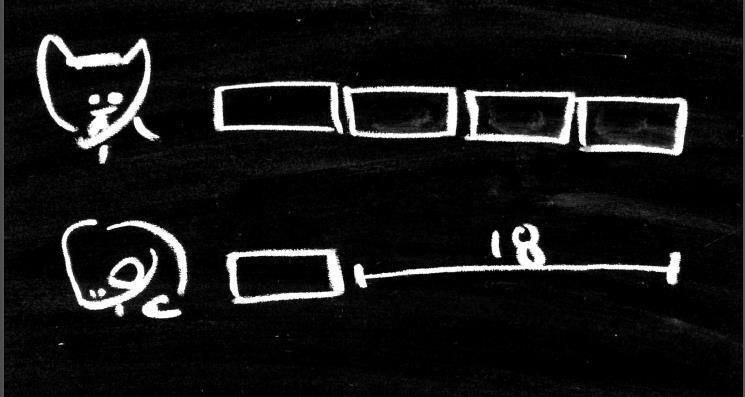One way to diagram relational information is to use bars, as demonstrated here. At this stage, the student may not know the quantity of each of the bars, but uses bars to represent an unknown quantity. The student is able to visualize that four times as many kittens were sold than lizards, and the difference between the quantities is 18.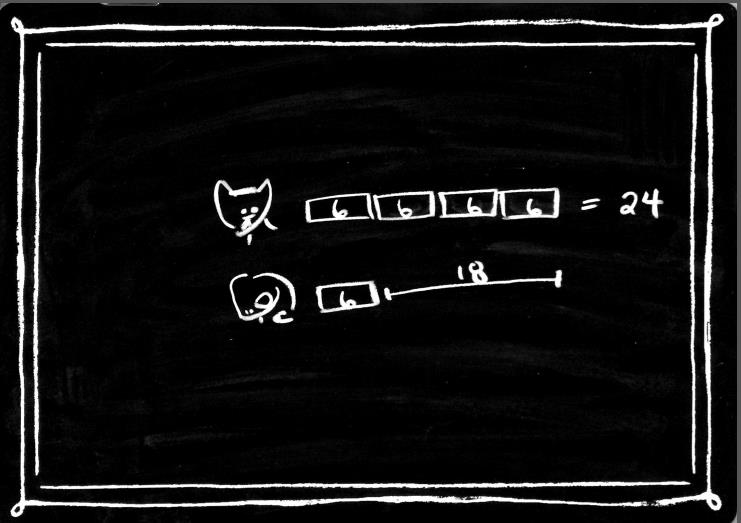Schematic

The student knows that because three of the bars represent 18 pets, each bar must represent six pets. The diagram, now completed, has helped the student visualize the question and also provided a visual strategy for solving the question.

These visualizations of unknown quantity can be a first step to understanding algebraic formulations.

Ahmed is participating in a 78-kilometre triathlon. He has to swim 3 kilometres. He has to bike four times as far as he has to run. How far will he travel by bike in the triathlon?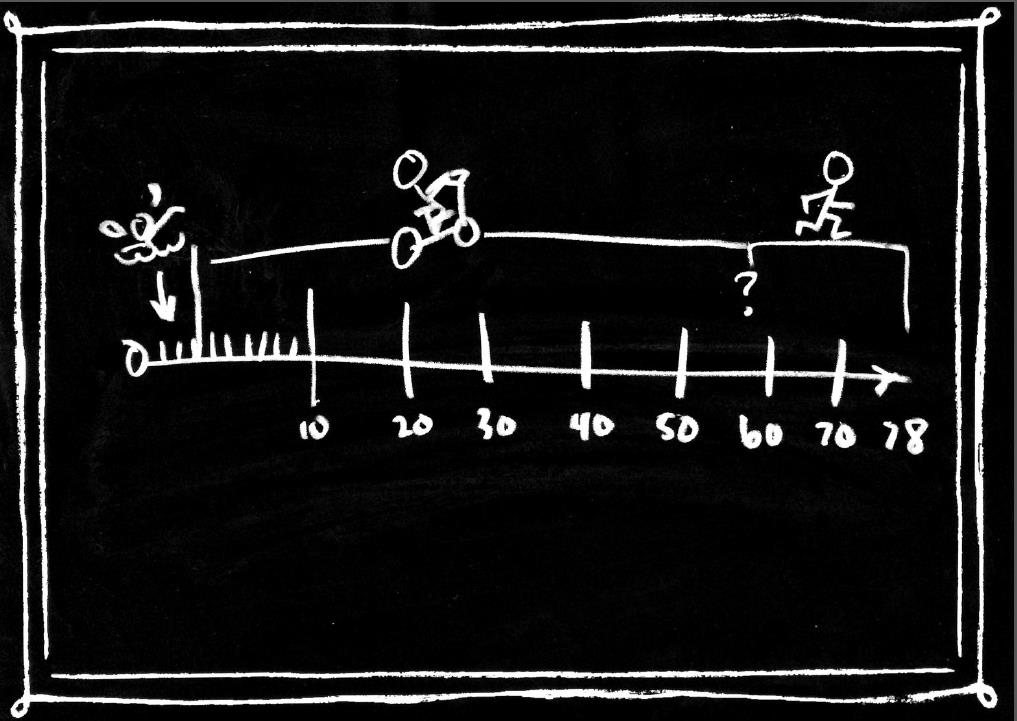Pictorial

For this word problem, the student drew a distance line because he recognized that distance is a relevant variable. Fortunately, the student was able to use the line to solve the first step of the problem (i.e., find the distance that is not swum), but gets stuck at this point. The student was not able to use the diagram to solve the second step of the problem (i.e., determine the distance that was biked).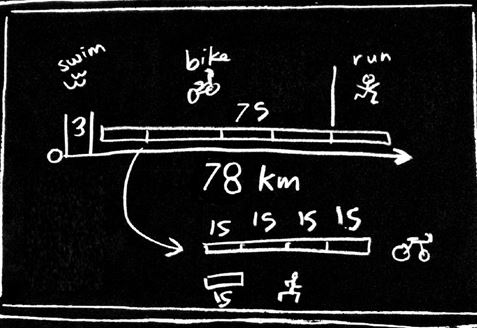Schematic

In this example, the student uses two types of visualization to solve the problem. Like the above answer, this student draws a line to show the swimming and non-swimming distances. For the second step of the problem, the student creates relational bars (at bottom of diagram) to show that the athlete biked four times as far as he ran, and the total distance was 75 kilometres. Once again, the diagram does more than visualize the problem; the diagram is a strategy for solving it.

Diagrams can be a valuable strategy when solving word problems, but more importantly, diagrams allow students to think and solve problems in new ways. For students with LDs, diagrams may help with organizing information, planning a solution, and implementing a solution—all processes they may find challenging! As a problem-solving strategy, diagram use is highly adaptable and dynamic, and it gives educators a great opportunity to monitor how students think and provide support where necessary. While teaching students to use diagrams effectively will take some time, the gains are lasting and the strategy may make all the difference for some students.

## References

Hutchinson, N. L. (1986). Instruction of representation and solution in algebraic problem solving with learning disabled adolescents (Unpublished doctoral dissertation). Simon Fraser University, Vancouver, BC.

Rosenzweig, C., Krawec, J., & Montague, M. (2011). Metacognitive strategy use of eighth-grade students with and without learning disabilities during mathematical problem solving: A think-aloud analysis. Journal of Learning Disabilities, 44, 508-520. doi: 10.1177/0022219410378445

Stylianou, D. (2010). Teachers’ conceptions of representation in middle school mathematics. Journal of Mathematics Teacher Education, 13, 325–434. doi: 10.1007/s10857-0109143-y.

Uesaka, Y., & Manalo, E. (2012). Task-related factors that influence the spontaneous use of diagrams in math word problems. Applied Cognitive Psychology, 26, 251–260. doi: 10.1002/acp.1816

van Garderen, D. (2007). Teaching students with LD to use diagrams to solve mathematical word problems. Journal of Learning Disabilities, 40, 540–553. doi:10.1177/00222194070400060501

van Garderen, D., & Montague, M. (2003). Visual-Spatial Representation, Mathematical Problem Solving, and Students of Varying Abilities. Learning Disabilities Research & Practice, 18, 246–254. doi:10.1111/1540-5826.00079

van Garderen, D., & Scheuermann, A. (2015). Diagramming word problems: A strategic approach for instruction. Intervention in School and Clinic, 50, 282–290. doi: 10.1177/1053451214560889

van Garderen, D., Scheuermann, A., & Jackson, C. (2012). Examining how students with diverse abilities use diagrams to solve mathematics word problems. Learning Disability Quarterly, 36 145–160. doi: 10.1177/0731948712438558

van Garderen, D., Scheuermann, A., & Poch, A. (2014). Challenges students identified with a learning disability and as high-achieving experience when using diagrams as a visualization tool to solve mathematics word problems. ZDM Mathematics Education, 46, 135–149. doi: 10.1007/s11858-013-0519-1

Zahner, D., & Corter, J. E. (2010). The process of probability problem solving: Use of external visual representations. Mathematical Thinking and Learning, 12, 177–204. doi: 10.1080/10986061003654240

## Related LD@school Resources

Click here to access the video, "Using Collaborative Teacher Inquiry to Support Students with LDs in Math", and the accompanying Viewers' Guide.

Click here to access the webinar, "Understanding Developmental Dyscalculia: A Math Learning Disability".

Click here to access the evidence-based article, "Evidence-Based Interventions for Students with Math Disabilities: One Size Does Not Fit All".

Click here to access the evidence-based article, "Concrete – Representational – Abstract: An Instructional Strategy for Math".

Click here to access the evidence-based article, "Explicit Instruction: A Teaching Strategy in Reading, Writing, and Mathematics for Students with Learning Disabilities".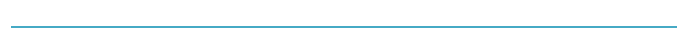Ian Matheson is a graduate student in the PhD program in Education at Queen's University with a focus in Learning and Cognition. Along with teaching and research experience at Queen's University, Ian also has experience as an occasional teacher with the Limestone District School Board in the elementary division.